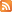Entries (RSS) -Comments (RSS)

The uncertainity principle by heisenbergTo get some clear understanding about the uncertainty principle, first, we need to know the quantum hypothesis.

Max Planck suggested that light, X-rays, and some other waves could not be emitted at the arbitrary rate, but in the form of energy packets called quanta.

According to the quantum hypothesis, each quantum--packet-- has certain amount of energy that was greater the higher the frequency of the waves. That implies that at higher frequencies, the emission of even a single quantum would require more energy than that was available. Thus the radiation at high frequencies gets reduced.

In order to predict the future position and velocity of a particle, one has to be able to determine its present position and velocity accurately. The only way to do this is to shine light on the particle and this will indicate its position. To determine more precisely one needs to use light of short wavelength(high frequency). Now, by Planck's quantum hypothesis, one cannot use an arbitrarily small amount of light; one has to use at least one quantum and this quantum will disturb the particle and change its velocity in a way that cannot be predicted. The more accurate one tries to measure the position, the greater will be the disturbance ( as it needs high energy quanta ). In other words, the more accurate one try to measure the position of a particle, the less accurate will be the speed and vice verse
This principle put an end to a notion(came from Newton's theory of gravity) that our universe is completely deterministic ( one can easily predict everything that would happen in the universe, if he knows its present state.)
It is only possible for the one who could observe the present state of universe with out disturbing it.

check this video.